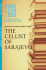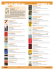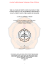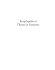# Kamala Markandaya`s Nectar in a Sieve

## Transcription

Kamala Markandaya`s Nectar in a Sieve
```Kamala
Markandaya's
Nectar
in a Sieve
BARRY ARGYLE
N E of the m a n y disputes, public, private, or e n t i r e l y
personal, f r o m w h i c h W . B . Y e a t s made his poetry
was t h a t w i t h T h o m a s M a n n . M a n n h a d w r i t t e n ,
" I n o u r t i m e the destiny of m a n presents its m e a n i n g i n
p o l i t i c a l t e r m s " ; a n d Y e a t s attached the r e m a r k to the
poem he w r o t e i n reply :
H o w can I, that g i r l s t a n d i n g there,
M y attention fix
O n R o m a n or on R u s s i a n
O r on S p a n i s h politics?
T h e poem has received little c o m m e n t :
i t needs no exegesis; a n d w h e n comment has been made, i t has been more
to do w i t h the poet as lascivious old m a n t h a n about the
poem as argument. B u t the argument is important, especi a l l y to o u r reading of l i t e r a t u r e f r o m newly independent
— t h o u g h often of course h i s t o r i c a l l y enormously old —
countries. M a n n ' s d i c t u m is more often heard, especially
i n those parts of the w o r l d such as N o r t h A m e r i c a where
the social sciences proliferate, each intent on u s i n g l i t e r ature as evidence of something else. S u c h a use, w h i l e i t
m a y help to elevate the social sciences, diminishes l i t e r ature — a l l literature, not just the poem, play o r novel
so used; so t h a t w h e n such c r i t i c s w r i t e of C h i n u a Achebe's
West A f r i c a n novels as great, we are beggared of language
w i t h w h i c h to describe Joseph Conrad's. O r i f K a m a l a
M a r k a n d a y a ' s Nectar in a Sieve is thought of as s i m p l y
another blow struck on behalf of p o l i t i c a l m a n , o u r attent i o n is distracted f r o m its organisation as a novel, the
sensibility t h a t i n f o r m s it, a n d the m o r a l intelligence that
controls t h a t sensibility.
36
BARRY ARGYLE
It is a short novel, so we read i t q u i c k l y . A h , yes, we
say, if we are inattentive or social scientists, the novel is
about the destruction of the t r a d i t i o n a l w a y of life i n an
I n d i a n village b y a tannery. Ergo : t r a d i t i o n a l r u r a l values,
w h i c h to minds still reared on w h a t they imagine Rousseau
wrote, are destroyed b y those of i n d u s t r i a l c a p i t a l i s m . Ind u s t r i a l capitalism, being western i n its origins, is w h i t e ;
thus the novel is about the w h i t e man's exploitation of the
non-white.
A t t h i s point, the novel h a v i n g served its
function as evidence of the c o m i n g revolution, r h e t o r i c
takes command.
B u t to ask w h a t the novel is about is a good place to
begin; for we are then t a l k i n g of the story, a n d our concern is thus w i t h the novel as literature. A n I n d i a n peasant w o m a n , p l a i n but intelligent, appears as a child, m a r ries, bears children, grows old, a n d prepares for death.
I n such a simple story, the plot — the causality that a r t i culates the story — is provided b y nature itself.
She
m a r r i e s a poor m a n because t h a t is u s u a l l y the fate of i l l favoured women w h o are themselves poor.
She bears
c h i l d r e n because t h a t is the fate of being a w o m a n ; she
grows old because t h a t is the fate of being h u m a n . L i f e
flourishes and dies, flourishes a n d dies; change is the only
absolute. T h i s fact is neither shallow n o r profound, but
s i m p l y a fact. It is also the condition i n w h i c h qualities
acquire meaning.
Once we are aware of t h i s fact:
that life is c i r c u l a r ,
not linear, we are i n a position to understand the organisat i o n of the novel. The novel does not begin w i t h the n a r rator's childhood, despite our s u m m a r y of the story as
t h o u g h it did. It begins w i t h R u c k m a n i , the n a r r a t o r , as
an old w o m a n r e c a l l i n g d u r i n g the day the experiences of
night :
Sometimes at night I t h i n k that m y husband is w i t h me
again, c o m i n g gently t h r o u g h the mists, and we are tranquil together. T h e n m o r n i n g comes, the w a v e r i n g grey
turns to gold, there is a s t i r r i n g w i t h i n me as the sleepers
awake, and he softly departs.
(p. 7; m y i t a l i c s ) .
KAMALA MARKANDAYA'S
NECTAR
37
T h e emphasis is on t i m e ; a n d as we read on we notice the
emphasis is sustained. E i g h t e e n of the novel's t h i r t y chapters contain i n t h e i r f i r s t sentence a reference to time, to
days, nights, years, seasons, to the festivals t h a t m a r k the
seasons. In the first sentence of each of the other twelve
chapters, there is a reference to journeys; w h i c h is to say,
to movement i n time. T h i s is not evidence of forgetfulness
or of a n impoverished i m a g i n a t i o n , but of the author's
constancy of concern w h i c h o u r s u m m a r y of the s t o r y i n dicates, a n d w h i c h the novel's last page confirms. R u c k m a n i returns, alone, to the f a m i l y w h i c h , on the first page,
stirs h e r to wakefulness.
B u t something more is going on i n the f i r s t p a r a g r a p h ;
it can be defined by our attending to the italicised adverbs
of manner.
"Gently," "tranquil," "softly":
i n terms of
organisation they are being used to establish b o t h mood and
character. T h e n a r r a t o r is speaking of the dawn, a t i m e
of calm, w h e n " T h e m o r n i n g steals upon the night, M e l t ing the d a r k n e s s . " T h e n a r r a t o r is also a n old woman,
now most alive w h e n asleep w i t h the m e m o r y of her dead
husband; to w h o m day means the t i m e w h e n others awake.
In retrospect, the description is seemly:
it conforms not
only to our imaginings, i f we are young, a n d to o u r recollections, i f we are old, but is also justified b y the n a r r a tor's experience as i t is explored i n the novel. Y e t i f we
carefully read the last page to w h i c h the f i r s t acts as postscript, we f i n d this :
T h e r e was a silence, I struggled to say w h a t h a d to be
said.
' D o not t a l k about it,' he said tenderly, 'unless y o u must.'
'It was a gentle passing,' I said. 'I w i l l t e l l y o u later.'
The n a r r a t o r as a n old w o m a n is t r y i n g to t e l l h e r son, to
whose care she has returned, of the m a n n e r of his father's
death. A s readers, we k n o w t h a t he died i n the r a i n on a
m u d d y b a n k after doing some of the lowliest w o r k possible, p i c k i n g stones i n a q u a r r y , to w h i c h poverty has
d r i v e n both h i m and his wife. I n social terms his death
38
BARRY ARGYLE
was wretched; but i n personal terms, i t was as he h a d
lived, gentle. The a u t h o r also ascribes this q u a l i t y to his
son, w h o answered " t e n d e r l y " ; a n d h e a r i n g the echo, we
are made aware that f r o m w h a t we have called the fact,
the condition of life, a q u a l i t y has acquired m e a n i n g : the
son has i n h e r i t e d his father's gentleness. O r to express
i t more abstractly, environment — w h i c h is the m a t r i x i n
w h i c h the protagonists live t h e i r d r a m a — is no more
powerful t h a n heredity. T h u s , l i k e the first, the second
p a r a g r a p h of the f i r s t page acquires new m e a n i n g :
P u l i is w i t h me because I tempted h i m , out of m y
desperation I l u r e d h i m a w a y f r o m his soil to mine.
Yet I have no fears now: w h a t is done is done,
there c a n be no r e p i n i n g .
W h e n we f i r s t read this, we are l u l l e d into a state of acquiescence: c'est la vie . . . T h e commonplace, " w h a t is done
is done" augurs a facile f a t a l i s m of the k i n d , f o r instance,
that we f i n d i n A h m e d A l i ' s Twilight
in Delhi:
m a n is
born, suffers, a n d dies. B u t w h e n w e have read the novel's
last page, we realise t h a t m a n also lives, and c a n l i v e well,
despite the odds of c i r c u m s t a n c e ; a n d t h i s is a cause for
celebration, as m u c h as circumstance is for desperation.
T h e r e can be " n o r e p i n i n g " because there is no need f o r it.
W h a t M a r k a n d a y a , then, is f o r c i n g us to do is to contemplate the opposites:
environment and heredity, f o r i n stance; or to look at the same i n f o r m a t i o n f r o m opposite
viewpoints. T h i s accounts for the shape of h e r novel. Since
T h o m a s M a n n ' s t i m e the h a b i t has been called i r o n y , a
" l o o k i n g - b o t h - w a y s " as he called i t ; but it is of course at
the heart of G e r m a n , Greek a n d H i n d u philosophy, a n y
Idealist philosophy i n fact. F o r the M a t e r i a l i s t , one lives
— f o r the State, the F u t u r e , or the W e l f a r e of the W o r l d ;
and one can live efficiently or inefficiently. T h e State,
the F u t u r e , or the W o r l d w i l l judge. T h e idea of l i v i n g
w e l l can be entertained only b y the Idealist, who, seeing
e x t e r n a l r e a l i t y before his eyes, can yet say i t does not
determine his values, t h o u g h i t m a y refine, sustain, con-
KAMALA MARKANDAYA'S NECTAR
39
f i r m or confound them. T r u t h a n d goodness, l i k e beauty
are i n the eye of the beholder.
A n understanding of this is essential to o u r appreciation
of Nectar in a Sieve. W i t h o u t i t we m a y respond, but we
w i l l not understand the nature of our response, to do w h i c h
is the task of the l i t e r a r y c r i t i c . W h e n , for instance, we
f i r s t read the t h i r d p a r a g r a p h , we are shocked:
"But I
a m comforted most w h e n I look at his hands. H e has no
fingers, o n l y stubs. . . ." Is this not a n example of that
complacency usually described, out of a profound ignorance,
or a careful editing, of h i s t o r y , as bourgeois? H e r e is a n
old w o m a n f i n d i n g comfort at the sight of a s m a l l boy
because he has no hands! S u c h callousness leaves us
dry-mouthed. N o r does w h a t follow mute o u r s h o c k :
. . . since w h a t has been t a k e n can never be given
back, but they are clean a n d sound. W h e r e the sores
were, there is now p i n k puckered f l e s h ; his l i m b s
are untouched. K e n n y a n d S e l v a m between t h e m have
kept m y promise to h i m .
T h e r e is not even a n adjective to persuade us to suspend
judgement; w h i l e the commonplace, " W h a t has been t a k e n
can never be given b a c k , " angers us because again i t displays t h a t easy acquiescence to w h a t is called F a t e .
Not
t i l l m u c h later do we realise t h a t P u l i , h a v i n g suffered
f r o m leprosy, is indeed l u c k y to have at least " s t u b s " left.
H e is not dead, o r even hopelessly crippled, only because
he, out of his desperation, allowed R u c k m a n i , out of hers,
to tempt h i m . H i s injuries, t h o u g h s t i l l a cause for sorrow,
are also a cause for joy, i n the same w a y t h a t the d i v i n i t y
of the s a c r i f i c i a l god is established only t h r o u g h his sacrifice, o r t h a t the w i s d o m of Socrates was confirmed only
i n the m a n n e r of his death. T h i s k i n d of i r o n y acquires
its apotheosis w h e n R u c k m a n i and N a t h a n , h e r husband,
meet P u l i for the f i r s t time. T h e y are destitute strangers
i n the t o w n P u l i knows as o n l y a scavenger c a n : " ' L e a d
on,' N a t h a n said. ' W e are i n y o u r hands.' "
Thus, we
are moved v i a p a r o n o m a s i a into metaphor, a q u a l i t y t h a t
R u c k m a n i ' s m u c h e a r l i e r m e n t i o n of " s o i l " now shares.
40
BARRY ARGYLE
P u l i ' s soil is barren, whereas hers is not. P u l i , as she
says, " t h e c h i l d I c l u n g to w h o was not mine, a n d he no
longer a c h i l d , " h a v i n g kept his promise to help her a n d
h e r husband, is alive and w e l l because her a c t u a l son,
Selvam, and his friend, K e n n y , a doctor, have kept h e r
promise to P u l i . T h u s not only are qualities l i k e gentleness inherited, but honour too; or, i n the poet W i l l i a m
E m p s o n ' s memorable line, they have learnt " a style f r o m
a despair."
The n a r r a t o r is aware of t h i s ; K e n n y , the w h i t e doctor,
denies i t : " T h e r e is no grandeur i n w a n t — or i n endurance." (p. 115) H e elaborates on it w h e n speaking to h e r :
'Acquiescent imbeciles,' he said scornfully, 'do y o u
t h i n k spiritual grace comes f r o m being i n want, o r f r o m
suffering? W h a t thoughts have you w h e n y o u r b e l l y is
empty or y o u r body s i c k ? T e l l m e they are noble ones
and I w i l l c a l l you a l i a r . '
'Yet our priests fast, and i n f l i c t on themselves
severe punishments, and we are taught to bear our
sorrows i n silence, and a l l this so that the
soul m a y be cleansed.'
H e struck his forehead. ' M y G o d ! ' he cried.
'I do not understand you. I never w i l l . Go, before
I too a m entangled i n y o u r philosophies.'
(p. 116)
T h i s w o u l d appear to be the voice of impatient, scientific
enlightenment condemning the b l i n d obscurantism of a
f a i t h that demeans. It w o u l d also seem to preclude a d i a logue between the two beliefs; c e r t a i n l y R u c k m a n i ' s attempt
at reply is cut short a n d she is f i n a l l y told to go. B u t as
readers, we are aware of a n i r o n y here, for the life of
suffering w h i c h the doctor condemns is the one he has h i m self chosen. B y rejecting, for w h a t e v e r reasons, the comforts of p r a c t i s i n g medicine i n the t o w n for the r i g o u r s of
r u r a l doctoring, he announces himself as already entangled
i n the philosophies he scorns. T o express the i r o n y i n
m o r e t r a d i t i o n a l terms, we see a g a i n the ancient conflict
between V i s h n u , the conservative principle of the universe,
the preserver of values, and S i v a , the destroying, but at
the same time the generative principle. W e are allowed
t h i s insight because, as readers, we share the author's
RAMALA MARKANDAYA'S NECTAR
41
B r a h m i n i c position. F r o m the careful placing, we are able
to create the pattern.
It is subtle. A s we have said, the story is about time,
w h i c h means change; but as the n a r r a t o r tells us, this i n
itself is not new:
C h a n g e I h a d k n o w n before, a n d it h a d been g r a d u a l .
M y father h a d been a h e a d m a n once, a person of
consequence i n our v i l l a g e : I h a d l i v e d to see h i m
r e l i n q u i s h this importance, but the a l t e r a t i o n w a s
so slow that w e h a r d l y k n e w w h e n it came. I h a d seen
both m y parents sink into old age a n d death, and here
too there was no violence.
(p. 29)
S u c h change partakes of the i n e v i t a b i l i t y , but g r a d u a l i n e v i t a b i l i t y of the seasons; a n d this a b i d i n g q u a l i t y of change
is sustained i n the novel t h r o u g h the comparison, w h i c h
acts m e t a p h o r i c a l l y :
W h i l e the sun shines on y o u and the fields a r e green
a n d beautiful to the eye, a n d y o u r husband sees beauty
i n y o u w h i c h no one has seen before, a n d y o u have a
good store of g r a i n l a i d a w a y for h a r d times, a roof
over y o u and a sweet s t i r r i n g i n y o u r body, w h a t more
can a w o m a n ask f o r ?
(p. 12)
L a t e r , i n a b a r r e n y e a r w h i c h has seen two of her sons
die, R u c k m a n i expresses h e r doubts to N a t h a n about the
f a m i l y ' s a b i l i t y even to harvest a good c r o p :
' Y o u w i l l see,' he said w i t h confidence. 'We w i l l
f i n d our strength. One look at the s w e l l i n g g r a i n
w i l l be enough to renew our vigour.'
Indeed, it did our hearts good to see the
paddy ripen. W e watched it as a dog watches a bone,
jealously, lest it be snatched a w a y ; or as a m o t h e r
h e r child, w i t h pride a n d affection. A n d most of a l l
w i t h fear.
(p. 97)
The n a r r a t o r is u s i n g the circumstances of experience as
the source of her metaphor; and for her i t remains a metaphor. T h e object is more i m p o r t a n t t h a n the t h i n g w i t h
w h i c h i t is compared. T h e crop is more i m p o r t a n t t h a n the
child, for instance. T h o u g h it w o u l d seem to those w h o b u y
t h e i r food i n a store that the l a n d a n d its crops are the
servants of men, to those w h o f a r m , it is they w h o serve
the l a n d : " T h e sowing of seed disciplines the body, a n d
42
BARRY ARGYLE
the s p r o u t i n g of the seed uplifts the s p i r i t . . ." (p. 107).
M o t h e r and c h i l d are the crop's servants, as are the dog
and its bone of a dead beast; for w i t h o u t the crop's good
favour none w i l l be fed. T h u s the wholeness of the interdependent w o r l d of the n a r r a t o r ' s experience is established.
A s readers, we can observe the r e a l i t y of language m i r r o r
this other ordered h i e r a r c h i c a l experience; for i f the metap h o r l i v e d too abundantly, d r a w i n g attention to itself, i t
w o u l d devour, unripe, the object it is meant to serve. T h u s
the a u t h o r has to m a i n t a i n a balance between the character
of her n a r r a t o r a n d the expectations of the reader.
She
does this as we have seen by a l l o w i n g language to become
metaphorical, but this is o n l y possible because she establishes i n a l l t h e i r detail those circumstances f r o m w h i c h
the n a r r a t o r ' s metaphors are d r a w n . T h u s , as w e l l as
c h i l d r e n that i n h e r i t t h e i r parents' f r u i t f u l
gentleness,
there are those b a r r e n years w h e n f i r s t R u c k m a n i a n d
then h e r daughter f a i l to conceive. A second w a y i n w h i c h
t h i s balance is m a i n t a i n e d is t h r o u g h m e t a p h o r becoming
symbol, w h i c h , l i k e the novel's i r o n y , we c a n appreciate
o n l y after we have completed o u r reading. T o r e t u r n to the
f i r s t page again, we read, " O n e by one they have come out
into the e a r l y m o r n i n g sunshine, m y son, m y daughter,
and P u l i . " In terms of experience t h i s is w h a t has happened:
n i g h t has departed, the f a m i l y awakes.
Metaphorically, the sunshine of the day brings the w a r m t h of
hope. S y m b o l i c a l l y , the c h i l d r e n follow t h e i r parents as
day follows night. T h e seasons, w h i c h are the condition
of the n a r r a t o r ' s experience, become the determinant of
The violence of social change is
matched b y the seasonal.
B u t the a u t h o r creates a still closer dependence; for the
more violent the seasons, the faster comes social change.
W h e n harvests are bad, f r o m flood o r drought, r u r a l f a m i lies can the more easily and ruthlessly be d r i v e n to w o r k i n
a tannery. T h i s done, the owners of the tannery can the
m o r e r e a d i l y b u y up the l a n d t h a t peasant farmers have
KAMALA MARKANDAYA'S NECTAR
43
been forced to leave, c o n f i r m i n g its barrenness i n the concrete that they spread over it. T h e hides on w h i c h it depends for its c o n t i n u i n g b i t t e r success now belong to h u m a n
beings. A l l of this the a u t h o r implies i n language at once
precise and metaphorical.
Y e t i f the tannery, t r a d i t i o n a l I n d i a n s y m b o l of social
baseness, is also the s y m b o l of twentieth c e n t u r y i n d u s t r i a l
rapacity, the hospital, "spruce and w h i t e , " is the s y m b o l
of its piety.
Its existence is justified not b y h i s t o r i c a l
a c c u r a c y — w h i c h is the concern of the h i s t o r i a n — but
b y m o r a l i n s i g h t : " n o t only money has b u i l t i t but men's
hopes a n d p i t y . " (p. 7) T h e author's c o n v i c t i o n appears
to be t h a t where there is hope, s u c h as K e n n y ' s , there is
also m o n e y ; and, too, where there is money, there c a n also
be hope; for w i t h o u t money, m e n w i l l die. Consequently
we recognise the reason for so m u c h emphasis i n the novel
on h o w m u c h things a n d services cost, are w o r t h , a n d w i l l
fetch i f sold. B y asserting t h i s t h r o u g h such detail, the
a u t h o r rejects the argument of p r i m i t i v e m y s t i c s w h o expect the E a s t to t u r n stone i n t o bread o n l y so t h a t its
death should live up t o t h e i r expectations. T h e result of
such a conviction, comparable w i t h Defoe's i n A
Journal
of the Plague Year a n d Moll Flanders, is t h a t the novel's
I n d i a n village w o r l d is f i r m t h r o u g h o u t :
. . . once or twice w h e n I saw the thickness of the
p u m p k i n vines I wondered nervously w h a t m i g h t lie
concealed there; and then I w o u l d take up k n i f e
and shovel to clear a w a y the tangle; but w h e n I
drew n e a r and s a w the broad glossy leaves a n d c u r l i n g
green tendrils I could not b r i n g m y s e l f to do i t ;
and now I a m g l a d I did not, for that same vine
yielded to me r i c h l y , p u m p k i n after p u m p k i n of a
size and colour that I never s a w elsewhere.
(p. 20)
B u t here again where the details are most precise, the
language assumes another d i m e n s i o n ; for the cause of
R u c k m a n i ' s nervousness is a snake t h a t h i d beneath the
vines u n t i l her husband k i l l e d it. It is e n t i r e l y credible
t h a t snakes should prefer the w a r m damp shady g r o u n d
that also produces the best p u m p k i n s ; this is a n observed
44
BARRY ARGYLE
fact of nature, and its observation helps establish the narr a t o r as a believable peasant, as does the fact that she
should fear the snake and her husband k i l l it, despite its
sacred significance. B u t just as p u m p k i n s are later referred to as "smooth-skinned . . . r o u n d a n d fleshed l i k e
y o u n g w o m e n , " so the snake's ancient significance, the
result of its secret dangerous ways, becomes attached to
people t h a t threaten a n d events t h a t are cruel. It fulfills
its role of symbol, but i t is also a necessary e v i l ; a n d e v i l
is sometimes the product of love and always the companion of happiness. T h e two aspects of existence, as the
a u t h o r sees it, exist side by side throughout the novel,
the i m m e d i a t e l y apparent m o o d of w h i c h is sombre while
the fortitude w i t h w h i c h R u c k m a n i and her f a m i l y face
life's c r u e l t y — the cause of t h i s sombreness — establishes
the deeper mood.
The success of a novel of this k i n d — one e m p l o y i n g a n
Idealist philosophy and shaped b y its demands — depends
on its p l a u s i b i l i t y . T h e p l a u s i b i l i t y of Nectar in a Sieve,
being told i n the f i r s t person, depends on characterisation.
F i r s t person n a r r a t i o n is always prone to i n c r e d i b i l i t y because the n a r r a t o r ' s point of view, uncorrected b y a n y
other, tends to prejudice. B y m a k i n g her a peasant, M a r k andaya goes some w a y to g u a r d i n g against t h i s : however
magnanimous we are, we condescend, a n d i n condescending, propose another point of view. Because the n a r r a t o r
is a peasant, we expect h e r commonplaces; but because she
is literate, we accept t h a t elucidation of the experience
f r o m w h i c h such commonplaces are distilled. B u t because
of the novel's shape, the p l a c i n g of comments, such as
K e n n y ' s , the language that grows f r o m metaphor to s y m bol, we are aware of the author's presence. A n d because
the ideas w i t h w h i c h she is dealing are profound and are
explored i n a novel that appears disguised as simple, we
recognise t h a t presence as a n intelligent a n d sophisticated
one. T h e tension between a u t h o r and n a r r a t o r that is
thereby set up is one of the novel's m a i n delights.
RAMALA MARRANDAYA'S
NECTAR
45
NOTE
1
R a m a l a M a r k a n d a y a , Nectar in a Sieve ( N e w Y o r k : J o h n Day,
1954; rpt. N e w Y o r k : Signet, n.d.). Subsequent references to
this edition appear i n the text.
ARIEL:
ISSUE
It has so f a r been the e d i t o r i a l policy of A R I E L to
devote at least one n u m b e r per year, u s u a l l y the t h i r d
number, to a special area of l i t e r a t u r e or to a special
author. It w o u l d therefore seem appropriate t h i s year
to devote V o l u m e 4, N u m b e r 3 (to be published i n J u l y
1973) to C a n a d i a n literature. A l r e a d y a n u m b e r of dist i n g u i s h e d C a n a d i a n c r i t i c s have promised to submit
articles for t h i s number. Since we should l i k e t h i s c r i t ical survey to be as comprehensive as possible we invite
articles for consideration on C a n a d i a n l i t e r a t u r e — even
at the r i s k of over-subscription.
W e invite also poems for consideration for this special
issue w h i c h w i l l contain a n e x t r a poetry section.
T h e deadline for submission is 1st M a y , 1973.
```

### Johnny Tremain by Esther Forbes Objectives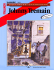### P1(表1） - Katawa Shoujo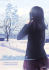### Spring 2010 Program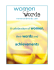### The Indie NEXT List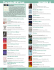### LA PEUPLADE Rights catalogue 2013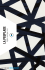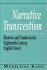### mlhs english summer reading 2013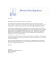### Invite AW.indd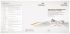### T1 - The Nicholson Place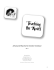### The Cellist of Sarajevo - Bookclub-in-a-Box